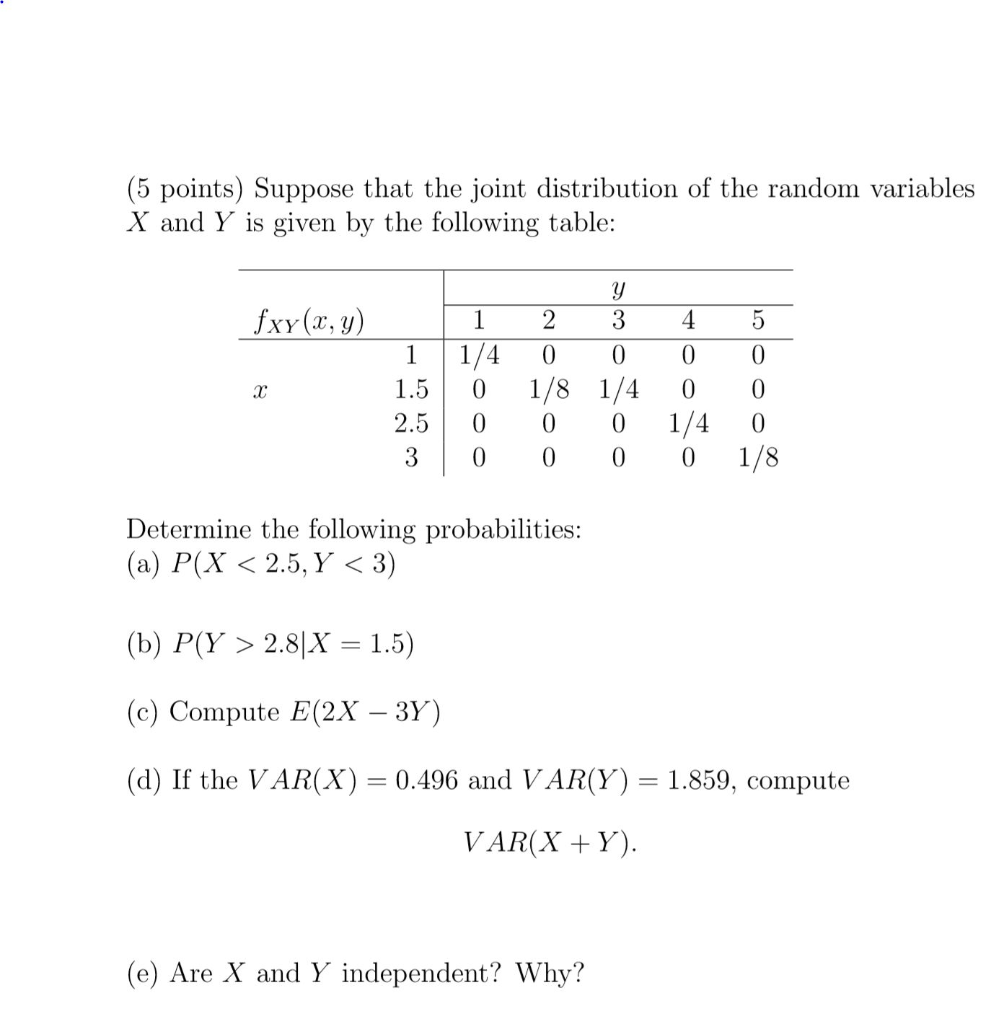# Solved: (5 Points) Suppose That The Joint Distribution Of The Random Variables X And Y Is Given By The Following Table: Fxy (x, Y) 2 4 1 3 1/4 1 0 0 1/8 1/4 1/4 1/8 1.5 0 0 2.5 0 0 0 0 0 0 Determine T

By |(5 points) Suppose that the joint distribution of the random variables X and Y is given by the following table: fxy (x, y) 2 4 1 3 1/4 1 0 0 1/8 1/4 1/4 1/8 1.5 0 0 2.5 0 0 0 0 0 0 Determine the following probabilities: (?) ?(X < 2.5, Y < 3) (b) ?(? 2.8|X 1.5) (c) Compute E(2X - 3Y) 0.496 and VAR(Y) = 1.859, compute (d) If the VAR(X) VAR(X Y) (e) Are X and Y independent? Why?